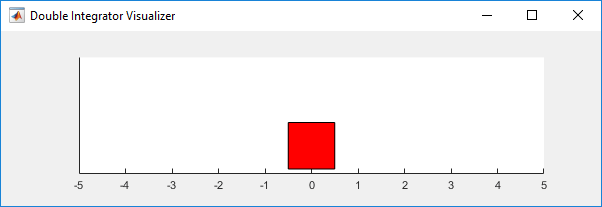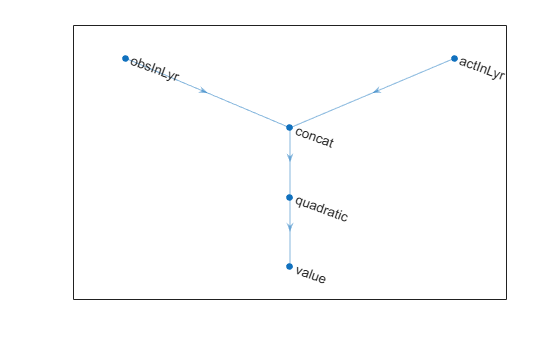Documentation

## Train DDPG Agent to Control Double Integrator System

This example shows how to train a deep deterministic policy gradient (DDPG) agent to control a second-order dynamic system modeled in MATLAB®.

For more information on DDPG agents, see Deep Deterministic Policy Gradient Agents. For an example that trains a DDPG agent in Simulink®, see Train DDPG Agent to Swing Up and Balance Pendulum.

### Double Integrator MATLAB Environment

The reinforcement learning environment for this example is a second-order system is a double integrator with a gain. The training goal is to control the position of a mass in a second-order system by applying a force input.For this environment:

• The mass starts at initial position of +/- 4 units.

• The force action signal from the agent to the environment is from `-`2 to 2 N.

• The observations from the environment are the position and velocity of the mass.

• The episode terminates if the mass moves more than `5 m` from the original position or if $|\mathit{x}|<0.01$

• The reward ${\mathit{r}}_{\mathit{t}}$, provided at every time step, is a discretization of $\mathit{r}\left(\mathit{t}\right)$:

`$\mathit{r}\left(\mathit{t}\right)=-\left({\mathit{x}\left(\mathit{t}\right)}^{\prime }\text{\hspace{0.17em}}\mathit{Q}\text{\hspace{0.17em}}\mathit{x}\left(\mathit{t}\right)+{\mathit{u}\left(\mathit{t}\right)}^{\prime }\text{\hspace{0.17em}}\mathit{R}\text{\hspace{0.17em}}\mathit{u}\left(\mathit{t}\right)\right)$`

where:

• $\mathit{x}$ is the state vector of the mass.

• $\mathit{u}$ is the force applied to the mass.

• $\mathit{Q}$ is the weights on the control performance. $\mathit{Q}=\left[10\text{\hspace{0.17em}}0;0\text{\hspace{0.17em}}1\right]$

• $\mathit{R}$ is the weight on the control effort. $\mathit{R}=0.01$

### Create Environment Interface

Create a predefined environment interface for the pendulum.

`env = rlPredefinedEnv("DoubleIntegrator-Continuous")`
```env = DoubleIntegratorContinuousAction with properties: Gain: 1 Ts: 0.1000 MaxDistance: 5 GoalThreshold: 0.0100 Q: [2x2 double] R: 0.0100 MaxForce: Inf State: [2x1 double] ```
`env.MaxForce = Inf;`

The interface has a continuous action space where the agent can apply force values from -`Inf` to `Inf` to the mass.

Obtain the observation and action information from the environment interface.

```obsInfo = getObservationInfo(env); numObservations = obsInfo.Dimension(1); actInfo = getActionInfo(env); numActions = numel(actInfo);```

Fix the random generator seed for reproducibility.

`rng(0)`

### Create DDPG agent

A DDPG agent approximates the long-term reward given observations and actions using a critic value function representation. To create the critic, first create a deep neural network with two inputs, the state and action, and one output. For more information on creating a neural network value function representation, see Create Policy and Value Function Representations.

```statePath = imageInputLayer([numObservations 1 1],'Normalization','none','Name','state'); actionPath = imageInputLayer([numActions 1 1],'Normalization','none','Name','action'); commonPath = [concatenationLayer(1,2,'Name','concat') quadraticLayer('Name','quadratic') fullyConnectedLayer(1,'Name','StateValue','BiasLearnRateFactor',0,'Bias',0)]; criticNetwork = layerGraph(statePath); criticNetwork = addLayers(criticNetwork,actionPath); criticNetwork = addLayers(criticNetwork,commonPath); criticNetwork = connectLayers(criticNetwork,'state','concat/in1'); criticNetwork = connectLayers(criticNetwork,'action','concat/in2');```

View the critic network configuration.

```figure plot(criticNetwork)```Specify options for the critic representation using `rlRepresentationOptions`.

`criticOpts = rlRepresentationOptions('LearnRate',5e-3,'GradientThreshold',1);`

Create the critic representation using the specified neural network and options. You must also specify the action and observation info for the critic, which you obtain from the environment interface. For more information, see `rlRepresentation`.

`critic = rlRepresentation(criticNetwork,obsInfo,actInfo,'Observation',{'state'},'Action',{'action'},criticOpts);`

A DDPG agent decides which action to take given observations using an actor representation. To create the actor, first create a deep neural network with one input, the observation, and one output, the action.

Construct the actor similarly to the critic.

```actorNetwork = [ imageInputLayer([numObservations 1 1],'Normalization','none','Name','state') fullyConnectedLayer(numActions,'Name','action','BiasLearnRateFactor',0,'Bias',0)]; actorOpts = rlRepresentationOptions('LearnRate',1e-04,'GradientThreshold',1); actor = rlRepresentation(actorNetwork,obsInfo,actInfo,'Observation',{'state'},'Action',{'action'},actorOpts);```

To create the DDPG agent, first specify the DDPG agent options using `rlDDPGAgentOptions`.

```agentOpts = rlDDPGAgentOptions(... 'SampleTime',env.Ts,... 'TargetSmoothFactor',1e-3,... 'ExperienceBufferLength',1e6,... 'DiscountFactor',0.99,... 'MiniBatchSize',32); agentOpts.NoiseOptions.Variance = 0.3; agentOpts.NoiseOptions.VarianceDecayRate = 1e-6;```

Then, create the DDPG agent using the specified actor representation, critic representation and agent options. For more information, see `rlDDPGAgent`.

`agent = rlDDPGAgent(actor,critic,agentOpts);`

### Train Agent

To train the agent, first specify the training options. For this example, use the following options:

• Run each training episode for at most 1000 episodes, with each episode lasting at most 200 time steps.

• Display the training progress in the Episode Manager dialog box (set the `Plots` option) and disable the command line display (set the `Verbose` option).

• Stop training when the agent receives an average cumulative reward greater than 195 over 10 consecutive episodes. At this point, the agent can balance the pendulum in the upright position.

For more information, see `rlTrainingOptions`.

```trainOpts = rlTrainingOptions(... 'MaxEpisodes', 5000, ... 'MaxStepsPerEpisode', 200, ... 'Verbose', false, ... 'Plots','training-progress',... 'StopTrainingCriteria','AverageReward',... 'StopTrainingValue',-66);```

The double integrator system can be visualized with `plot(env)` during training or simulation.

`plot(env)`Train the agent using the `train` function. This is a computationally intensive process that takes several hours to complete. To save time while running this example, load a pretrained agent by setting `doTraining` to `false`. To train the agent yourself, set `doTraining` to `true`.

```doTraining = false; if doTraining % Train the agent. trainingStats = train(agent,env,trainOpts); else % Load pretrained agent for the example. load('DoubleIntegDDPG.mat','agent'); end```### Simulate DDPG Agent

To validate the performance of the trained agent, simulate it within the double integrator environment. For more information on agent simulation, see `rlSimulationOptions` and `sim`.

```simOptions = rlSimulationOptions('MaxSteps',500); experience = sim(env,agent,simOptions);````totalReward = sum(experience.Reward)`
```totalReward = single -65.9933 ```